# JP4664427B2 - Distance calculation device - Google Patents

## Info

Publication number
JP4664427B2
JP4664427B2 JP2009214522A JP2009214522A JP4664427B2 JP 4664427 B2 JP4664427 B2 JP 4664427B2 JP 2009214522 A JP2009214522 A JP 2009214522A JP 2009214522 A JP2009214522 A JP 2009214522A JP 4664427 B2 JP4664427 B2 JP 4664427B2
Authority
JP
Japan
Prior art keywords
angle
object
distance
moving
moving object
Prior art date
Legal status (The legal status is an assumption and is not a legal conclusion. Google has not performed a legal analysis and makes no representation as to the accuracy of the status listed.)
Active
Application number
JP2009214522A
Other languages
Japanese (ja)
Other versions
JP2009300457A (en
Inventor

Original Assignee

Priority date (The priority date is an assumption and is not a legal conclusion. Google has not performed a legal analysis and makes no representation as to the accuracy of the date listed.)
Filing date
Publication date
Application filed by 富士通株式会社 filed Critical 富士通株式会社
Priority to JP2009214522A priority Critical patent/JP4664427B2/en
Publication of JP2009300457A publication Critical patent/JP2009300457A/en
Application granted granted Critical
Publication of JP4664427B2 publication Critical patent/JP4664427B2/en
Application status is Active legal-status Critical
Anticipated expiration legal-status Critical

## Images

•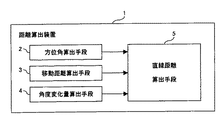•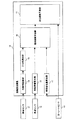••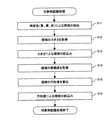•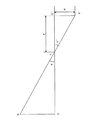•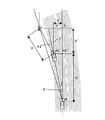•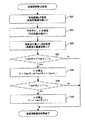•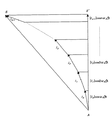•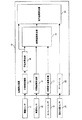•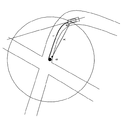••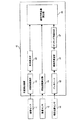•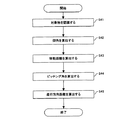•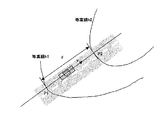•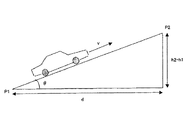••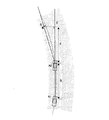•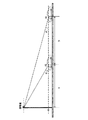••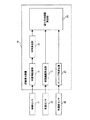•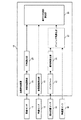•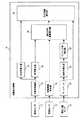•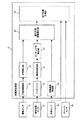••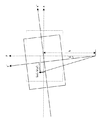•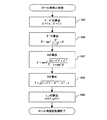•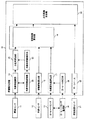•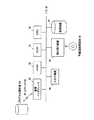## Description

The present invention relates to a method for calculating a distance between a moving object and a fixed object, and more specifically, for example, a vehicle state quantity such as an elevation angle and an azimuth angle between a traveling vehicle and an object, a vehicle speed, and a yaw rate. The present invention relates to a distance calculation device and a calculation program for calculating an accurate distance between a vehicle traveling on a road and an object.

Car navigation technology that guides the driving path to the driver according to the current position of the vehicle and the destination by developing the position of the vehicle using a map database or GPS (Global Positioning System) has been developed and widely spread. . In such a system, based on the latitude and longitude information obtained by GPS, the position of the host vehicle is displayed superimposed on a map, and the distance to the intersection is calculated.
As an example of such a prior art, there is the following patent document.

Japanese Patent No. 3381212 (P3388131) “Navigation Device”

This patent document recognizes an object whose position can be specified by map information, and uses the travel distance of the vehicle between two points in time and the elevation angle from each point to the same object to determine the object and the subject vehicle. A technique for calculating the distance between the vehicles and correcting the position of the host vehicle is disclosed.

However, this conventional technique can only calculate the straight distance to the traffic light, and there is an error when calculating the actual distance on the road, such as when there is no curved road or traffic light in the traveling direction of the vehicle. There was a point.

In view of the above problems, the problem of the present invention is a distance having a highly accurate distance measurement function by properly using an azimuth angle, a speed or acceleration (pitching angle), a yaw rate, etc., including an elevation angle from a vehicle with respect to an object. To provide a calculation device and a distance calculation program.

FIG. 1 is a block diagram showing the principle configuration of a distance calculation apparatus according to the present invention. The figure is a block diagram of the principle configuration of a distance calculating device according to a first embodiment described later. The distance calculating device 1 according to the first embodiment includes at least an azimuth calculating means 2, a moving distance calculating means 3, an angle change amount calculating means 4, And a linear distance calculation means 5.

The azimuth calculating means 2 calculates an angle formed by a moving object, for example, a direction connecting a traveling vehicle and a fixed object, for example, a traffic light, and a moving direction of the moving object on a horizontal plane as an azimuth. The distance calculating means 3 calculates the moving distance of the moving object between two time points, for example, 100 ms as the processing interval for calculating the linear distance.

The angle change amount calculation means 4 calculates a rotation angle around the vertical axis passing through the center of gravity of the moving object between the two time points as an angle change amount in the traveling direction, and the linear distance calculation means 5 calculates the azimuth angle. The linear distance between the moving object and the object is calculated using the outputs of the means 2, the moving distance calculating means 3, and the angle change amount calculating means 4.

In an embodiment of the invention, a current position sensor for detecting a current position of a moving object, a database means for outputting map information including roads around the current position according to an output of the current position sensor, a database means, an azimuth angle The distance calculation device 1 may further include a travel distance calculation unit that calculates a travel distance from the current position to the target object using outputs of the calculation unit 2 and the straight line distance calculation unit 5.

In the embodiment, an object recognition unit that recognizes an object from an image including the object and gives the recognition result, for example, the coordinates of the object to the azimuth angle calculation unit 2 can be further provided. In this case, the object recognition monitoring means for monitoring whether or not the output of the object recognition means deviates from a predetermined range, and when the deviation is detected, the output of the object recognition means is corrected to An object recognition correcting means for giving to the angle calculating means can be further provided.

Alternatively, the rotational angle around the moving direction of the moving object can be determined by using the output of the speed sensor that gives the speed of the moving object to the moving distance calculating means 3, the current position sensor, the database means, and the database means and the speed sensor. A roll angle calculating unit that calculates the roll angle; and a roll angle correcting unit that corrects the recognition result of the object, for example, coordinates using the roll angle and the recognition result of the object, and gives the azimuth calculating unit 2 with the roll angle. You can also.

Further, in the embodiment, the rotational angular velocity around the vertical axis of the moving object is detected, and the yaw rate sensor given to the angle change amount calculating means 4 and the outputs of the yaw rate sensor and the linear distance calculating means 5 are used to detect the moving object from the moving object. A distance predicting means for predicting the distance to the object may be further provided, and the output of the yaw rate sensor and / or the change range of the output deviates from the predetermined output range and / or the output change range. A yaw rate monitoring means for monitoring whether or not, and a yaw rate correction means for correcting the output of the yaw rate sensor using a past yaw rate or speed value when a deviation is detected, and giving it to the angle change amount calculation means 5 It can also be provided.

Next, a distance calculation apparatus corresponding to Example 3 of the present invention includes at least an elevation angle calculation means, a movement distance calculation means, a pitching angle calculation means, and a movement direction distance calculation means.
The elevation angle calculation means calculates the angle formed by the direction connecting the moving object and the target with the horizontal plane as the elevation angle, and the pitching angle calculation means calculates the moving object on the plane determined by the moving direction of the moving object and the vertical direction. The rotation angle is calculated as the pitching angle, and the movement distance calculation means calculates the movement distance between the two time points of the moving object as in the first embodiment whose principle is explained in FIG. Then, the moving direction distance calculating means calculates the moving direction component (of the vehicle) of the linear distance from the moving object to the target using the outputs of the elevation angle calculating means, the moving distance calculating means, and the pitching angle calculating means.

In the embodiment of the invention, similarly to the first embodiment, the present position sensor, the database means, and the movement distance calculation means can be further provided.
Further, in the embodiment, it is possible to further include an object recognition unit that recognizes the object from an image including the object and gives the recognition result to the elevation angle calculation unit. And an object recognition correcting means.

In this case, a speed sensor, a current position sensor, a database unit, a roll angle calculation unit, and a roll angle correction unit similar to those described above may be further provided. The roll angle correction unit corrects the recognition result of the object. The correction result is given to the elevation angle calculation means.

Furthermore, in the embodiment, an acceleration sensor that detects acceleration in the moving direction of the moving object is further provided, and the pitching angle calculation means can also calculate the pitching angle using the output of the acceleration sensor.

Alternatively, in addition to the current position sensor and database means similar to those described above, the apparatus further comprises an inclination angle calculating means for calculating an inclination angle in the moving direction near the current position of the moving object using the output of the database means, and a pitching angle calculating means However, the pitching angle can be calculated using the output of the tilt angle calculating means, or further provided with an acceleration sensor, and the pitching angle calculating means uses the output of the acceleration sensor in addition to the output of the tilt angle calculating means. The pitching angle can also be calculated.

As described above, according to the present invention, the azimuth of the moving object, the amount of change in the traveling direction of the moving object, or the like, or the elevation angle of the moving object, the pitching angle of the moving object, etc. The straight line distance and the moving direction distance of the moving object are calculated.

It is a principle block diagram of the distance calculation device of the present invention. 1 is a configuration block diagram of Embodiment 1 of a distance calculation device of the present invention. 3 is a flowchart for overall processing of distance calculation in the first embodiment. It is a detailed flowchart of a target object recognition process. It is explanatory drawing of the azimuth calculation method. It is explanatory drawing of the straight line distance calculation method. It is a detailed flowchart of a straight line distance calculation process. It is explanatory drawing of a travel distance prediction method. It is a block diagram of the configuration of Example 2 of the distance calculation device. It is explanatory drawing of the travel distance calculation method. It is a detailed flowchart of a mileage calculation process. It is a structure block diagram of Example 3 of a distance calculation apparatus. 12 is an overall flowchart of a traveling direction distance calculation process according to the third embodiment. It is explanatory drawing of the driving | running | working state of the vehicle for demonstrating inclination-angle calculation. It is explanatory drawing of the inclination-angle calculation method. It is a detailed flowchart of an inclination angle calculation process. It is a figure which shows the driving | running | working condition of the vehicle for demonstrating the advancing direction distance calculation. It is explanatory drawing of the advancing direction distance calculation method. It is a detailed flowchart of the advancing direction distance calculation process. It is a structure block diagram of Example 4 of a distance calculation apparatus. It is a block diagram of the configuration of Example 5 of the distance calculation device. It is a structure block diagram of Example 6 of a distance calculation apparatus. It is a block diagram of the configuration of Example 7 of the distance calculation device. It is a block diagram of the configuration of Example 8 of the distance calculation device. It is explanatory drawing of the roll angle correction method. It is a detailed flowchart of a roll angle correction process. It is a block diagram of the configuration of Example 9 of the distance calculation device. It is a figure explaining loading to the computer of the program of this invention.

The embodiment of the present invention will be described in detail below by dividing it into several examples.
In the present invention, as a fixed object for which, for example, a linear distance is calculated from a moving object, for example, a traveling vehicle, an image can be captured by a camera installed in the traveling vehicle, including a traffic light and a road sign. Distances to various objects such as signs, railroad crossings, footbridges, pedestrian crossings, and stop lines are calculated. In order to calculate the distance, data is taken from the image sensor, the yaw rate sensor, the vehicle speed sensor, the acceleration sensor, etc., for example, every 100 ms, and various processes such as object recognition and azimuth calculation are performed every 100 ms. For example, the linear distance to the object is calculated every 100 ms. In the following description, an embodiment will be described using a traffic light as a specific example of an object.

FIG. 2 is a block diagram of the configuration of the first embodiment of the distance calculation apparatus of the present invention. In the figure, the output of the image sensor 11, the vehicle speed sensor 12, and the yaw rate sensor 13 is given to the distance calculation device 10, respectively. As the image sensor 11, for example, a CCD camera or a CMOS camera is used, and the camera is installed in front of the vehicle in order to photograph the traveling direction, and its optical axis is basically the same as the traveling direction of the vehicle. Installed. The vehicle speed sensor 12 detects the speed of the wheels of the vehicle, and is composed of, for example, four sensors respectively installed on four wheels. The yaw rate sensor 13 detects the rotational angular velocity of the vehicle around the vertical axis through the center of gravity of the vehicle.

The object recognition unit 14 inside the distance calculation device 10 uses the image captured by the image sensor 11 to recognize the object, for example, recognize the coordinates of the object. As described above, a traffic signal is taken as an example of the object, and a method for recognizing the traffic signal will be described later with reference to FIG. The azimuth angle calculation unit 15 calculates the azimuth angle of an object recognized by the object recognition unit 14, for example, a traffic light. That is, the angle formed by the projection line of the line connecting the object recognized as the center of gravity of the vehicle and the traveling direction of the vehicle on the horizontal plane passing through the center of gravity of the vehicle is calculated as the azimuth angle.

The travel distance calculation unit 16 calculates the travel distance of the vehicle per 100 ms using the output of the vehicle speed sensor 12. The angle change amount calculation unit 17 uses the output of the yaw rate sensor 13 to calculate the rotation angle of the vehicle around the vertical rotation axis during the processing interval of 100 ms as the angle change amount. When the angular velocity of the yaw rate sensor 13 is ω (rad / s), the angle change amount is 0.1 ω (rad).

Here, the straight line distance calculation unit 18 calculates a straight line distance between the vehicle and the object using the outputs of the azimuth angle calculation unit 15, the movement distance calculation unit 16, and the angle change amount calculation unit 17. This calculation method will be described later with reference to FIGS. The travel distance prediction unit 19 predicts the travel distance from the current point of the vehicle to the target point using the outputs of the straight line distance calculation unit 18 and the yaw rate sensor 13. The calculation method will be described later with reference to FIG.

FIG. 3 is an overall process flowchart of distance calculation in the first embodiment. When the processing is started in the figure, first, in step S1, the position of the object is recognized using the output of the image sensor 11, for example, the coordinates of the red and blue signals of the traffic light are output in the image, and in step S2. An angle formed by the traveling direction of the vehicle and the direction toward the object, that is, an azimuth angle is calculated, and the moving distance of the vehicle between two time points using the output of the vehicle speed sensor 12 in step S3, that is, a processing interval of 100 ms. In step S4, the angle change amount around the vertical axis of the vehicle is calculated using the output of the yaw rate sensor 13.

However, step S1 to step S4 can be executed independently of each other, and the order thereof can be changed or processed in parallel. Subsequently, in step S5, a linear distance to the object is calculated using the calculated azimuth angle, moving distance, and angle change amount, and in step S6, the calculation result of the linear distance and the output of the yaw rate sensor 13 are used. The travel distance is predicted, and the process ends.

FIG. 4 is a detailed flowchart of the object recognition processing by the object recognition unit 14 of FIG. When the processing is started in the figure, a region having a specific color, here, a blue, yellow, and red color of a signal is detected in step S11. Consider extracting the color area of the signal that is lit, but since it is not known which color is lit, it is assumed here that each of the three color areas is detected. For each of the three colors, a plurality of areas may be detected, or no area may be detected.

The size of the region extracted in step S12, here, the size of all the regions extracted by the three colors, is acquired, respectively, and depending on the distance to the traffic light in step S13, The area is narrowed down by a size having a set sufficient range.

Subsequently, in step S14, the perimeter of each region, here, all the regions extracted in step S13, is acquired. In step S15, the circularity of each region is determined using the perimeter length l and the area S of the region. Calculated. The circularity is calculated by 4πS / l 2 , and step S16
Thus, only when the area is narrowed down by the size of the circularity, that is, when the value of the circularity is larger than a predetermined threshold, for example, 0.8, the area is recognized as the signal light area of the traffic light. Then, as the processing result, the coordinates of the center of the signal light area of the traffic light in the image are output. Here, the circularity is 1 for a complete circle, but here, the coordinates of the center of the region where the circularity is larger than the threshold among all the regions extracted in step S13 are output. It is conceivable that a plurality of coordinates are output. In this case, the azimuth angle is calculated for each of the output coordinates.

FIG. 5 is an explanatory diagram of an azimuth calculation method by the azimuth calculation unit 15. This figure is also used for the calculation of the elevation angle, which will be described later, but in the calculation of the azimuth angle, the x-coordinate on the image, that is, on the horizontal plane passing through the position L of the camera lens, Calculations are performed using the x coordinate.

In FIG. 5, the position of the traffic light on the horizontal plane is S, and when a perpendicular line is dropped from S to the plane perpendicular to the optical axis direction of the lens, the foot is O, the focal length of the lens is F, and the position of the imaging surface is O. ', Where the x coordinate of the traffic light (signal light) in the image is x (pixel unit) and the size of the pixel in the x direction is l, the azimuth angle α to be obtained is equal to α', and the azimuth angle is tan −1 (xl / F).

FIG. 6 is an explanatory diagram of a method for calculating the distance X from the vehicle to the object S by the linear distance calculation unit 18. As shown in FIG. 6, it is assumed that the position of the traffic light is S, and the vehicle moves from the A1 point to the A2 point during the time Δt. The azimuth angle of the traffic light from the A1 point is θ 1 , and the azimuth angle of the traffic light from the A2 point is θ 2 . A vertical line is dropped from A2 in the direction of travel of the vehicle at A1, and the intersection is
2 ′. If the distance of A1-A2 ′ is D ′ and the distance of A2-A2 ′ is d, the movement distance D calculated by the movement distance calculation unit 16 and the angle change amount γ calculated by the angle change amount calculation unit 17 When D ′ and d are approximately expressed,
D ′ = D cos γ
d = Dsinγ
A perpendicular line is drawn from S in the traveling direction at A1, and the intersection is defined as O. If the distance from the point A2 ′ to O is X ′, the following equation is established.

d + X ′ tan (θ 2 + γ) = (X ′ + D ′) tan θ 1
Solving for X ′, X ′ = (D′ tan θ 1 −d) / (tan (θ 2 + γ) −tan θ 1 )
= (Dcos γ tan θ 1 −D sin γ) / (tan (θ 2 + γ) −tan θ 1 )
The distance X from A2 to S is represented by the following equation.

X = X ′ / cos (θ 2 + γ)
FIG. 7 is a flowchart of a straight line distance calculation process from the vehicle to the target by the straight line distance calculation unit 18. In the figure, when the process is started, the movement distance D is calculated by the movement distance calculation unit 16 in step S20, the azimuth angles θ 1 and θ 2 are calculated by the azimuth angle calculation unit 15 in step S21, and the angle change amount calculation unit 17 is calculated in step S22. the value of γ is set by whether or not the absolute value of the calculation equation the denominator of X 'in the step S23 is a threshold t 1 that is larger is determined. When the denominator is 0, the value of X ′ cannot be obtained, and even when the denominator is close to 0, X ′ cannot be obtained accurately. In such a case, the process is immediately terminated.

If the threshold t 1 greater than the value of X 'is calculated in step S24, step S25
In is determined whether the threshold t 2 is greater than or not there is a denominator calculation formula of X is immediately terminated process is not greater, the value of X and the process is terminated calculated in step S26 in greater .

If the absolute value of the denominator of X ′ is less than or equal to the threshold t 1 and the value of X ′ cannot be calculated in step S23, the past distance between the vehicle and the object, the subsequent vehicle speed, yaw rate, etc. are used. Thus, the current position of the vehicle can be predicted, and the linear distance to the object can be calculated. In FIG. 6, when the distance from the point A1 to the traffic light S is X ″, X ′ is given by the following equation, and it is also possible to calculate X by replacing the aforementioned X ′ with this value.

X ′ = X ″ cos θ 1 −D ′
When the denominator of X in the step S25 of FIG. 7 is the threshold value t 2 less is not able to calculate the X, are slow extreme, when there is not much movement distance, a value of D from the value of X " It is possible to subtract and obtain the value of X approximately.

FIG. 8 is an explanatory diagram of a method for predicting the travel distance from the current position to the object by the travel distance prediction unit 19. The travel distance prediction unit 19 predicts the distance from the current position of the vehicle to the object along the travel path from the past yaw rate and the speed of the vehicle. In this prediction, the value of the yaw rate as the rotational angular velocity around the vertical axis passing through the center of gravity of the vehicle on the travel path from the current position A to the position S of the object is constant, and the absolute value of the vehicle speed is also constant. As shown in FIG. 8, the vectors l 0 , l 1 , l 2 ... Are predicted every minute time Δt, and the calculation is repeated until the sum of the vectors reaches the position S of the object. The total distance is taken to predict the distance traveled to the object.

In FIG. 8, when the foot of the perpendicular line from the position of the object with respect to the current traveling direction from the current point A of the vehicle is S ′, the angle formed by the first vector l 0 with the direction of S ′ is the angular velocity ω And the minute time Δt. The angle formed by the next vector l 1 and the S ′ direction is the product of 2ω and the minute time Δt. Similarly, the direction of each vector is obtained, and the length of each vector and its direction are determined by calculating the length of each vector as the product of the current vehicle speed v and the minute time Δt. The travel distance until the position S is reached is predicted.

Next, a second embodiment of the present invention will be described. FIG. 9 is a block diagram illustrating a configuration of the distance calculating device according to the second embodiment. Comparing this figure with the first embodiment, a travel distance calculation unit 23 is provided instead of the travel distance prediction unit 19, and the output of the azimuth angle calculation unit 15 and the linear distance calculation unit 18 are provided for the travel distance calculation unit 23. The output of the database 22 using the output of the current position sensor 21 outside the distance calculation device 10 is given.

The current position sensor 21 detects the current position of the vehicle, and is, for example, a GPS (Global Positioning System) generally used in a car navigation system. Although it is possible to obtain the absolute position such as the latitude, longitude, altitude, etc. of the current position of the vehicle by GPS, since this position generally includes a certain amount of error, these positions are taken into account for the error. It is necessary to refer to

The database 22 is map information indicating the shape of the road (elevation and radius of curvature), the position of an object such as a traffic light, a pedestrian bridge, and a railroad crossing, and is displayed on the map using the position of the host vehicle detected by the current position sensor 21. The position of the vehicle can be specified. The database 22 provides the travel distance calculation unit 23 with, for example, data on an object near the host vehicle, road shape data, and the like.

Based on the position of the object obtained from the map information in the database 22, the travel distance calculation unit 23 uses the distance between the object calculated by the linear distance calculation unit 18 and the host vehicle, the direction to the object, A point at which the angle formed with the traveling direction of the vehicle is equal to the azimuth angle calculated by the azimuth angle calculation unit 15 is determined as the position of the host vehicle, and the distance on the road from the position to the object is calculated from the map information To do. This calculation method will be described with reference to FIG. 10 and FIG.

FIG. 11 is a flowchart of the travel distance calculation process. First, in step S31, as shown in FIG. 10, a circle whose radius is the linear distance between the host vehicle and the object is drawn on the map with the position of the object S as the center. In step S32, the circumference of the circle is changed. Such arcs on the road, four arcs in FIG. 10, are detected, and the length l of each arc is calculated in step S33.

Subsequently, in step S34, when considering the end of each arc, that is, the point moving in the counterclockwise direction on the circle in FIG. 10, from the position where the point first reaches each arc, the length of ¼ on each arc is reached. The position is detected as a candidate position of the vehicle. That is, here, the road is one lane above and below, and the center position of the left lane is detected as a candidate vehicle position.

Subsequently, in step S35, an angle formed by a line indicating the traveling direction of the vehicle at the candidate position of the vehicle, that is, a line parallel to the tangential direction of the road, and a line connecting the candidate position of the vehicle and the object is set for each arc. The candidate position of the vehicle that is calculated and is the closest to the azimuth value calculated by the azimuth angle calculation unit 15 among the four values calculated in step S36, in FIG. 10, is determined as the current position of the vehicle. In step S37, the distance from the current position of the vehicle to the object is calculated along the road shape, and the process ends.

Next, Embodiment 3 of the present invention will be described with reference to the block diagram of FIG. In the same figure, the distance calculation device 10 is similar to the second embodiment described with reference to FIG. 9, the object recognition unit 14 using the output of the image sensor 11, the movement distance calculation unit 16 using the output of the vehicle speed sensor 12, and the current position sensor. In addition to the database 22 using the output of 21, the elevation angle calculation unit 25 that calculates the elevation angle of the object direction using the output of the object recognition unit 14, and the inclination angle that calculates the vehicle inclination angle using the output of the database 22 The calculation unit 26 includes a pitching angle calculation unit 27 that calculates the pitching angle of the vehicle using the output of the inclination angle calculation unit 26, and the traveling direction distance calculation unit 28 instead of the linear distance calculation unit 18 includes an elevation angle calculation unit 25, Using the outputs of the movement distance calculation unit 16 and the pitching angle calculation unit 27, the vehicle traveling direction component of the linear distance to the object is calculated.

The elevation angle calculation method by the elevation angle calculation unit 25 is basically the same as the azimuth angle calculation method described in FIG. However, the elevation angle is calculated using the coordinate in the x-axis direction and the focal length F of the lens in addition to the coordinate in the y-axis direction perpendicular to the horizontal plane including the traveling direction of the vehicle on the imaging surface in FIG. Done. That is, if the x and y coordinates of the traffic light (signal light) at the point S ′ in FIG. 5 are x and y, the value of the elevation angle is obtained using the dimension l in the x and y directions of the pixel.

Sought by.
The inclination angle calculation unit 26 receives map data around the current position detected by the current position sensor 21 from the database 22, and based on the difference in elevation between two points whose elevation values are known around the current position. The inclination angle of the vehicle surrounding position is calculated and given to the pitching angle calculation unit 27. Details of the inclination angle calculation method will be described later.

The pitching angle calculation unit 27 indicates the pitching angle of the vehicle, that is, the pitch between the axis connecting the front and rear of the vehicle and the horizontal line, using the inclination angle calculated by the inclination angle calculation unit 26, that is, the inclination angle in the traveling direction of the road. The angle is calculated. When the pitching angle of the vehicle changes, the elevation angle changes, making it impossible to determine the exact distance to the object. Therefore, it is necessary to calculate the pitching angle and correct the elevation angle. The elevation angle correction is performed by the traveling direction distance calculation unit 28 with respect to the elevation angle calculated by the elevation angle calculation unit 25.

The relationship between the vehicle pitching angle and the road inclination angle is determined by the expansion and contraction of the front, rear, left and right suspensions of the vehicle, and the expansion and contraction of the suspension is determined by the spring coefficient. Therefore, in this embodiment, the relationship between the tilt angle and the vehicle pitching angle is experimentally obtained. The pitching angle is measured when the vehicle is stopped on a horizontal surface and when the vehicle is stopped on a slope having an inclination angle θ. By measuring the pitching angle by changing the tilt angle θ, the relationship between the tilt angle and the pitching angle can be experimentally determined. When there is no measurement result of the pitching angle with respect to the tilt angle, the pitching angle is calculated by interpolating the measurement result.

FIG. 13 is an overall flowchart of the traveling direction distance calculation process according to the third embodiment. When the process is started in the figure, the object recognition unit 14 first recognizes the position of the object in the same manner as in FIG. 4, calculates the elevation angle in the direction toward the object in step S42, and moves the moving distance in step S43. In step S44, the pitching angle is calculated using the output of the inclination angle calculation unit 26. In step S45, a vector representing the distance from the current position of the vehicle to the object is a component parallel to the traveling direction of the vehicle. The travel direction distance is calculated, and the process ends.

The calculation of the tilt angle will be described. FIG. 14 and FIG. 15 are explanatory diagrams of a tilt angle calculation method, and FIG. 16 is a flowchart of the tilt angle calculation process. FIG. 14 is an explanatory diagram of the running state of the vehicle on the map output from the database 22. Around the current position of the vehicle,
It is assumed that there are two points P1 and P2 whose altitude values are known.

FIG. 15 is an explanatory diagram of a method for calculating the tilt angle θ. As described with reference to FIG. 14, if the difference in elevation while the vehicle travels from point P1 to P2 is h2−h1 and the distance between points P1 and P2 on the map is d, the inclination angle θ is expressed by the following equation: Given.

θ = tan −1 {(h2−h1) / d}
When the process is started in the flowchart of the inclination angle calculation process of FIG. 16, first, in steps S51 and S52, two points P1 and P2 that are closest to the current position of the host vehicle and have known altitude values are searched. In step S53, the distance d on the map between the two points is calculated. In step S54, the inclination angle θ is calculated, and the process ends.

Next, calculation of the traveling direction distance will be described with reference to FIGS. FIG. 17 is an explanatory diagram of a traveling state of the vehicle. In the figure, it is assumed that the vehicle has moved from point A1 to point A2 by a distance D during a processing time, for example, 100 ms. Assuming that the vehicle continues to travel in a straight line direction, the perpendicular foot drawn from the position S of the object in the traveling direction is S ′, and the distance X from point A2 to S ′ is calculated as the traveling direction distance. To do. It is assumed that the vehicle actually approaches the object S on a curved road from the point A2.

FIG. 18 is a diagram illustrating a state in which the traveling state of the vehicle is viewed from the side. As shown in FIG. 18, it is assumed that the vehicle has moved from point A1 to point A2 during time Δt. The elevation angle of the traffic light from the point A1 is φ 1 , and the elevation angle of the traffic light from the point A2 is φ 2 . When the movement distance calculated by the movement distance calculation unit 16 from the A1 point to the A2 point is D and the component parallel to the traveling direction of the vehicle of the distance from the A2 point to the traffic light is X, the following equation is established.

(X + D) tanφ 1 = Xtanφ 2
Solving for X,
X = Dtanφ 1 / (tanφ 2 −tanφ 1 )
Thus, the distance to the object can be calculated. Even if the optical axis of the camera is facing up only elevation [rho c than in the traveling direction of the vehicle (set at the time of camera installation), the distance X is expressed by the following equation.

X = Dtan (φ 1 + ρ c ) / (tan (φ 2 + ρ c ) −tan (φ 1 + ρ c ))
Further, the pitching angle calculated by the pitching angle calculation unit 27 at the point A1 is represented by ρ 1 ,
The pitching angle calculated by the pitching angle calculating unit 27 at the point A2 and [rho 2. At this time, the distance X is expressed by the following equation.

X = Dtan (φ 1 + ρ c + ρ 1 ) / (tan (φ 2 + ρ c + ρ 2 ) −tan (φ 1 + ρ c + ρ 1 ))
As described above, the traveling direction distance calculation unit 28 is based on the elevation angle calculated by the elevation angle calculation unit 25, the movement distance calculated by the movement distance calculation unit 16, and the pitching angle calculated by the pitching angle calculation unit 27. The component parallel to the traveling direction of the vehicle at the distance of is calculated.

When the advancing direction distance calculation process in FIG. 19 is started, the movement distance D is calculated by the movement distance calculation unit 16 in step S56, the two elevation angles are calculated by the elevation angle calculation unit 25 in step S57, and the pitching angle calculation unit 27 is calculated by step S58. one of the values of the pitching angle is set, the threshold value t 1 is greater than or not there is the absolute value of the denominator of the formula for calculating the value of X at step S59, it is determined, immediately ends processing when t 1 is the following values If the threshold value is exceeded, the value of X is calculated in step S60 and the process ends. When the absolute value of the denominator of the equation for calculating X in step S59 is 0 or close to 0, X cannot be calculated accurately, but when the vehicle speed is extremely slow and the moving distance D is small, the previous one The traveling direction distance X can also be obtained approximately by subtracting the movement distance D from the distance X calculated at the time of

Next, a fourth embodiment of the present invention will be described. FIG. 20 is a configuration block diagram of a distance calculation apparatus according to the fourth embodiment. Compared to the third embodiment of FIG. 12, the acceleration sensor 30 for detecting the acceleration in the front-rear direction of the vehicle, that is, the traveling direction, is provided outside the distance calculation device 10 in place of the current position sensor 21, and the pitching angle. The difference is that the calculation unit 27 calculates the pitching angle using the output of the acceleration sensor and gives it to the traveling direction distance calculation unit 28. In FIG. 20, an acceleration sensor 30 is installed, for example, in the center of the vehicle, and detects an acceleration component in the longitudinal direction of the vehicle by a piezoelectric element or the like. The pitching angle calculation unit 27 calculates the pitching angle from a known relationship between the acceleration as the output of the acceleration sensor 30 and the pitching angle of the vehicle.

The relationship between the vehicle pitching angle and the acceleration depends on the expansion and contraction of the suspension, that is, the spring coefficient, as in the relationship with the tilt angle. For this reason, the relationship between the acceleration and the pitching angle is obtained experimentally.

That is, the pitching angle is measured when the vehicle is stopped on a horizontal surface and when the vehicle is traveling on the horizontal surface at a certain acceleration. By changing the value of acceleration and measuring the pitching angle, the relationship between the acceleration and the pitching angle is experimentally determined. When the pitching angle with respect to the acceleration value detected by the acceleration sensor 30 has not been measured, the pitching angle is obtained by interpolating the measurement result.

Next, Example 5 of the present invention will be described. FIG. 21 is a block diagram illustrating the configuration of the distance calculation device according to the fifth embodiment. Comparing this figure with FIG. 12 showing the third embodiment and FIG. 20 showing the fourth embodiment, all the components of these embodiments are provided, and the pitching angle calculation unit 27 outputs the inclination angle calculation unit 26 to the output. In addition, the only difference is that the pitching angle is calculated using the output of the acceleration sensor 30 and given to the traveling direction distance calculation unit 28.

The pitching angle calculation unit 27 in FIG. 21 calculates the pitching angle based on an experimental relationship between the pitching angle, the road inclination angle, and the acceleration. Pitching when tilt angle and acceleration are given by measuring the pitching angle when the vehicle is traveling on a slope with a certain tilt angle at a constant acceleration, and changing the tilt angle and acceleration. The corner can be calculated. Alternatively, using the experimental relationship between the pitching angle and the inclination angle described in the third embodiment and the relationship between the pitching angle and the acceleration described in the fourth embodiment, the pitching angles are respectively obtained and the sum thereof is obtained. By calculating, it is also possible to calculate the pitching angle corresponding to the tilt angle and acceleration.

FIG. 22 is a configuration block diagram of Embodiment 6 of the distance calculation device. 12 is different from the third embodiment in FIG. 12 in that an azimuth calculation unit 15 and a travel distance calculation unit 23 are further provided. The azimuth calculation unit 15 calculates the azimuth of the vehicle in the same manner as in the first embodiment of FIG.

The travel distance calculation unit 23 calculates the travel distance using the output of the azimuth angle calculation unit 15, the output of the database 22, and the output of the traveling direction distance calculation unit 28. In the second embodiment described with reference to FIG. 9, the travel distance calculation unit 23 uses the output of the linear distance calculation unit 18, that is, the linear distance from the position of the vehicle to the object, to calculate the travel distance along the road to the object. Calculated. This is illustrated in FIG. On the other hand, the traveling direction distance calculation unit 28 in FIG. 22 calculates the distance X in the traveling direction of the vehicle as described in FIG. 17, and the linear distance to the current position of the vehicle and the object is the azimuth angle calculating unit 15. Is easily calculated by using ∠SA 2 S ′. Therefore, by using this linear distance, the travel distance calculating unit 23 can calculate the travel distance to the object.

FIG. 23 is a block diagram illustrating a configuration of the distance calculating device according to the seventh embodiment. 12 is different from the third embodiment described in FIG. 12 in that the yaw rate sensor 13 described in the first embodiment in FIG. Here, the travel distance prediction unit 19 replaces the calculation result of the linear distance calculation unit 18 in FIG. 2, that is, the calculation result of the traveling direction distance calculation unit 28, that is, FIG. The travel distance to the target object is predicted using the distance X described in (1) in the same manner as the method described in FIG.

FIG. 24 is a block diagram showing the configuration of the eighth embodiment of the distance calculating apparatus according to the present invention. 2 is compared with the first embodiment of FIG. 2, a current position sensor 21 is provided outside the distance calculation device 10, and a database 22 that receives the output of the current position sensor 21 inside the device 10, and an output of the database 22 The roll angle calculation unit 32 that calculates the roll angle of the vehicle using the output of the vehicle speed sensor 12, that is, the angle at which the vehicle rotates about the axis in the traveling direction, the object recognition unit 14, and the azimuth calculation The error of the recognition result by the object recognition unit 14 generated by the generation of the roll angle is corrected using the output of the object recognition unit 14 and the output of the roll angle calculation unit 32. The difference is that a roll angle correction unit 33 is provided to give the coordinates of the object of the correction result to the azimuth angle calculation unit 15.

The roll angle calculation unit 32 shown in FIG. 24 generally calculates a roll angle that occurs when the vehicle is traveling on a curve. When this roll angle occurs, the recognition result by the object recognition unit 14 becomes inaccurate, that is, the elevation angle and the azimuth angle change due to the rotation of the optical axis of the image sensor 11, and the accurate distance to the object is determined. It becomes impossible to seek. Therefore, it is necessary to calculate the roll angle, correct the recognition result by the object recognition unit 14, and correctly calculate the azimuth angle.

The roll angle is determined by the curvature radius of the road on which the vehicle is traveling and the expansion and contraction of the suspension. The relationship between the roll angle and the radius of curvature of the road is calculated experimentally, and the roll angle when a road with a constant radius of curvature travels at a constant speed is measured. By varying the value of the radius of curvature and the speed and measuring the value of the roll angle, the relationship between the roll angle and the radius of curvature of the road and the speed of the vehicle can be determined experimentally.

The roll angle correction unit 33 corrects the recognition result of the object recognition unit 14 using the roll angle calculated by the roll angle calculation unit 32, for example, the coordinate position on the center image of the signal light of the traffic light. FIG. 25 is an explanatory diagram of a roll angle correction method. In order to correct the inclination of the coordinate axis due to the roll angle θ, the coordinate (x, y) is calculated from the coordinate (x ′, y ′).

As shown in FIG. 25, the roll angle is θ, the coordinates of the object (x ′, y ′), the height from the center of gravity of the vehicle to the center of the camera is L, the pixel size of the camera is l, and the corrected object Let (x, y) be the coordinates of. l 'is used to replace x' and y 'with distances X' and Y 'from the center of the image.

X ′ = x ′ · l, Y ′ = y ′ · l,
The following two formulas are established according to the relationship between X and Y and X ′ and Y ′.
(L + Y) 2 + X 2 = (L + Y ′) 2 + X ′ 2

When this is solved for X and Y, the following equation is obtained.

Finally, the camera pixel size l is used to return to the coordinate system on the image as in the following equation.
x = X / l, y = Y / l
FIG. 26 is a flowchart of the roll angle correction process. In the figure, distances X ′ and Y ′ from the center of the image of the object are calculated from the coordinates (x ′, y ′) of the object when the roll angle θ occurs in step S65, and θ in step S66. 'Is calculated, the distance X from the center of the image of the corrected object is calculated in step S67, Y is calculated in step S68, and the coordinates (x, y) on the corrected image are calculated in step S69. To finish the process.

Finally, Example 9 of the present invention will be described. FIG. 27 is a configuration block diagram of Embodiment 9 of the distance calculation device. Compared to FIG. 2, for example, the first embodiment of FIG. 2, a travel distance calculation unit 23 is provided in place of the travel distance prediction unit 19, and in addition to the output of the linear distance calculation unit 18 for the travel distance calculation unit 23 Then, the output of the database 22 based on the output of the azimuth calculation unit 15 and the output of the current position sensor 21 is given, and the object recognition unit 14 monitors whether or not the recognition result of the object recognition unit 14 is abnormal. The monitoring unit 35 and the object recognition correction unit 36 that corrects the recognition result of the object recognition unit 14 and supplies it to the azimuth angle calculation unit 15 when there is an abnormality. Similarly, the output (angular velocity) or output of the yaw rate sensor 13 The yaw rate sensor monitoring unit 38 that monitors the rate of change (angular acceleration) of the sensor and the yaw rate that is given to the angle change amount calculation unit 17 by correcting the output of the yaw rate sensor when the output or the rate of change of the output is abnormal That it includes a Tadashibu 39 is different.

In FIG. 27, the object recognition unit 14 recognizes an object based on an image captured by the image sensor 11, but there is a possibility that an error occurs in the recognition result due to various causes. Therefore, when it is determined that there is an abnormality in the recognition result of the target object, for example, when the position of the traffic signal as the target object is significantly deviated during the processing interval of 100 ms, compared to the past recognition result, the target object The recognition monitoring unit 35 notifies the object recognition correction unit 36 of the abnormality.

When the object recognition correction unit 36 is notified that there is an abnormality in the recognition result of the object recognition unit 14, the object recognition correction unit 36 obtains the result of the prediction using the linear prediction or the Kalman filter based on the past object recognition result. The correction result of the object recognition is given to the azimuth calculation unit 15.

The yaw rate sensor monitoring unit 38 detects an angular velocity change that is difficult to consider as a normal movement of the vehicle, such as when the output of the yaw rate sensor 13, that is, the value of the rotational angular velocity around the vertical axis of the vehicle output by the yaw rate sensor suddenly changes. When this is done, this is notified to the yaw rate correction unit 39.

The yaw rate correction unit 39 performs linear prediction based on the past yaw rate or speed value, instead of the output value of the yaw rate sensor, when the output of the yaw rate sensor 13 or an abnormality in its change rate is notified from the yaw rate sensor monitoring unit 38. Further, the yaw rate value corrected using the prediction result by the Kalman filter is given to the angle change amount calculation unit 17.

In FIG. 27, for example, an object recognition monitoring unit 35, an object recognition correction unit 36, a yaw rate sensor monitoring unit 38, and a yaw rate correction unit 39 are added as compared with the second embodiment of FIG. However, for example, on the basis of the seventh embodiment of FIG. 23, each of these units is added, and it is naturally possible to employ a configuration in which the object recognition correction unit 36 corrects the recognition result and supplies the correction result to the elevation angle calculation unit 25.

Although the details of the distance calculation apparatus and calculation program of the present invention have been described above, the distance calculation apparatus can naturally be configured as a general computer system. FIG. 28 is a block diagram showing the configuration of such a computer system, that is, a hardware environment.

In FIG. 28, the computer system includes a central processing unit (CPU) 50, a read only memory (ROM) 51, a random access memory (RAM) 52, a communication interface 53, a storage device 54, an input / output device 55, and a portable storage medium reading device. 56, and a bus 57 to which all of them are connected.

As the storage device 54, various types of storage devices such as a hard disk and a magnetic disk can be used, and FIGS. 3, 4, 7, 11, 13, and 16 are stored in the storage device 54 or the ROM 51. 19, the program shown in the flowchart of FIG. 19 and the like, the program of claims 16 to 19 of the claims of the present invention, and the like are stored and executed by the CPU 50, Distance calculation using an image sensor, a vehicle speed sensor, a current position sensor, an acceleration sensor, a yaw rate sensor, or the like in this embodiment is possible.

Such a program is stored in, for example, the storage device 54 from the program provider 58 via the network 59 and the communication interface 53, or stored in a portable storage medium 60 that is commercially available and distributed. It can also be set in the reading device 56 and executed by the CPU 50. As the portable storage medium 60, various types of storage media such as a CD-ROM, a flexible disk, an optical disk, a magneto-optical disk, and a DVD can be used. A program stored in such a storage medium is read by the reader 56. By being read, it is possible to calculate an accurate distance from the traveling vehicle to the object in the present embodiment.

As described above in detail, according to the present invention, for example, an object such as a traffic light is recognized, the azimuth angle or elevation angle from the own vehicle to the object is calculated, and the yaw rate and traveling direction of the vehicle are calculated as necessary. It is possible to accurately calculate the distance between the host vehicle and the object by correcting the influence of the acceleration, the rotation angle around the axis in the traveling direction, and the pitching angle due to the inclination angle of the road surface. Further, the travel distance to the object can be calculated by using the travel state of the vehicle and the map data. Further, when the purpose is to automatically stop the vehicle, the distance to the stop line can be accurately calculated using the stop line as an object, and the distance to various objects can be calculated according to the purpose.
(Appendix 1)
In an apparatus for calculating the distance between a moving object and a fixed object,
Azimuth angle calculating means for calculating an angle formed by a direction connecting the moving object and the target object and a moving direction of the moving object on a horizontal plane as an azimuth angle;
A moving distance calculating means for calculating a moving distance between two time points of the moving object;
Angle change amount calculating means for calculating a rotation angle of the moving object around the vertical axis passing through the center of gravity of the moving object between the two time points as an angle change amount in the moving direction;
A linear distance calculating means for calculating a linear distance between the moving object and the object using outputs of the azimuth calculating means, the moving distance calculating means, and the angle change amount calculating means; Calculation device.
(Appendix 2)
A current position sensor for detecting a current position of the moving object;
Database means for outputting map information including roads around the current position in response to the output of the current position sensor;
The apparatus further comprises travel distance calculating means for calculating a distance on the moving trajectory from the current position to the object using outputs of the database means, the azimuth angle calculating means, and the straight line distance calculating means. The distance calculation apparatus according to 1.
(Appendix 3)
The distance calculation apparatus according to claim 1, further comprising an object recognition unit that recognizes an object from an image including the object and gives the recognition result to the azimuth angle calculation unit.
(Appendix 4)
Object recognition monitoring means for monitoring whether the output of the object recognition means deviates from a predetermined range;
The distance according to claim 3, further comprising: an object recognition correction unit that corrects an output of the object recognition unit and gives the azimuth calculation unit when the deviation is detected by the object recognition monitoring unit. Calculation device.
(Appendix 5)
A current position sensor for detecting a current position of the moving object;
Database means for outputting map information including roads around the current position in response to the output of the current position sensor;
A speed sensor that gives the speed of the moving object to the moving distance calculating means;
Roll angle calculation means for calculating a rotation angle about the moving direction axis of the moving object as a roll angle using outputs of the database means and the speed sensor;
The apparatus further comprises roll angle correction means that corrects the coordinates as the recognition result of the object using the outputs of the roll angle calculation means and the object recognition means, and gives them to the azimuth angle calculation means. The distance calculation device according to attachment 3.
(Appendix 6)
A yaw rate sensor that detects a rotational angular velocity around the vertical axis of the moving object and provides the angular change amount calculating means;
The distance calculation apparatus according to claim 1, further comprising distance prediction means for predicting a distance on a moving trajectory from a moving object to the target object using outputs of the yaw rate sensor and the linear distance calculation means. .
(Appendix 7)
A yaw rate sensor monitoring means for monitoring whether the output value of the yaw rate sensor and / or the rate of change of the output value deviates from a predetermined range of output values and / or a range of the rate of change of output values;
When the yaw rate sensor monitoring means detects the deviation, yaw rate correction means for correcting the output value of the yaw rate sensor based on the past output of the yaw rate sensor and the value of the speed of the moving object and giving the output to the angle change amount calculating means. The distance calculation apparatus according to appendix 6, further comprising:
(Appendix 8)
In an apparatus for calculating the distance between a moving object and a fixed object,
An elevation angle calculating means for calculating, as an elevation angle, an angle formed by a direction connecting the moving object and the object with the horizontal plane;
A moving distance calculating means for calculating a moving distance between two time points of the moving object;
A pitching angle calculating means for calculating the rotation angle of the moving object on the plane determined by the moving direction and the vertical direction of the moving object as a pitching angle;
And a moving direction distance calculating unit that calculates a moving direction component of a linear distance from the moving object to the target using outputs of the elevation angle calculating unit, the moving distance calculating unit, and the pitching angle calculating unit. Distance calculation device.
(Appendix 9)
A current position sensor for detecting a current position of the moving object;
Database means for outputting map information including roads around the current position in response to the output of the current position sensor;
An azimuth angle calculating means for calculating an angle formed by a direction connecting the moving object and the target object and a moving direction of the moving object on a horizontal plane as an azimuth angle;
It further comprises travel distance calculation means for calculating a distance on a moving trajectory from the current position to the object using outputs of the database means, azimuth angle calculation means, and movement direction distance calculation means. The distance calculation apparatus according to appendix 8.
(Appendix 10)
9. The distance calculating apparatus according to claim 8, further comprising: an object recognizing unit that recognizes the object from an image including the object and gives the recognition result to the elevation angle calculating unit.
(Appendix 11)
A current position sensor for detecting a current position of the moving object;
Database means for outputting map information including roads around the current position in response to the output of the current position sensor;
A speed sensor that gives the speed of the moving object to the moving distance calculating means;
Roll angle calculating means for calculating a rotation angle around the moving direction of the moving object as a roll angle using outputs of the database means and the speed sensor;
Supplementary note 10 further comprising: a roll angle correction unit that corrects coordinates as a recognition result of an object using outputs of the roll angle calculation unit and the object recognition unit and gives the coordinate to the elevation angle calculation unit. The described distance calculation device.
(Appendix 12)
A current position sensor for detecting a current position of the moving object;
Database means for outputting map information including roads around the current position in response to the output of the current position sensor;
An inclination angle calculating means for calculating an inclination angle of the moving direction near the current position of the moving object using the output of the database means;
9. The distance calculation apparatus according to claim 8, wherein the pitching angle calculation means calculates the pitching angle using the output of the tilt angle calculation means.
(Appendix 13)
An acceleration sensor for detecting acceleration in the moving direction of the moving object;
13. The distance calculating apparatus according to appendix 12, wherein the pitching angle calculating means calculates the pitching angle using the output of the acceleration sensor in addition to the output of the tilt angle calculating means.
(Appendix 14)
An acceleration sensor for detecting acceleration in the moving direction of the moving object;
The distance calculating apparatus according to appendix 8, wherein the pitching angle calculating means calculates the pitching angle using the output of the acceleration sensor.
(Appendix 15)
A yaw rate sensor that detects a rotational angular velocity around the vertical axis of the moving object and provides the angular change amount calculating means;
An azimuth angle calculating means for calculating an angle formed by a horizontal plane between a direction connecting the moving object and the target object and a moving direction of the moving object as an azimuth angle;
The apparatus further includes a distance predicting unit that predicts a distance on a moving trajectory from the moving object to the target object using outputs of the yaw rate sensor, the azimuth calculating unit, and the moving direction distance calculating unit. 9. The distance calculation device according to 8.
(Appendix 16)
In a program used by a computer that calculates the distance between a moving object and a fixed object,
A procedure for calculating, as an azimuth angle, an angle formed on a horizontal plane between a direction connecting the moving object and the target and the moving direction of the moving object;
A procedure for calculating a moving distance between two time points of the moving object;
A procedure for calculating a rotation angle of the moving object around the vertical axis passing through the center of gravity of the moving object between the two time points as an angle change amount in the moving direction;
A distance calculation program for causing a computer to execute a procedure for calculating a linear distance between a moving object and an object using the calculated azimuth angle, moving distance, and angle change amount.
(Appendix 17)
In a storage medium used by a computer that calculates the distance between a moving object and a fixed object,
Calculating an angle formed on a horizontal plane between a direction connecting the moving object and the target and the moving direction of the moving object as an azimuth;
Calculating a moving distance between two time points of the moving object;
Calculating a rotation angle of the moving object about the vertical axis passing through the center of gravity of the moving object between the two time points as an angle change amount in the moving direction;
A computer-readable portable type storing a program for causing a computer to execute a step of calculating a linear distance between a moving object and an object using the calculated azimuth angle, moving distance, and angle change amount Storage medium.
(Appendix 18)
In a program used by a computer that calculates the distance between a moving object and a fixed object,
A procedure for calculating an angle formed by the direction connecting the moving object and the target and the horizontal plane as an elevation angle;
A procedure for calculating a moving distance between two time points of the moving object;
A procedure for calculating the rotation angle of the moving object on the plane determined by the moving direction and the vertical direction of the moving object as a pitching angle;
A distance calculation program for causing a computer to execute a procedure for calculating a moving direction component of a linear distance from a moving object to a target object using the calculated elevation angle, moving distance, and pitching angle.
(Appendix 19)
In a storage medium used by a computer that calculates the distance between a moving object and a fixed object,
Calculating an angle formed by the direction connecting the moving object and the target and the horizontal plane as an elevation angle;
Calculating a moving distance between two time points of the moving object;
Calculating a rotation angle of the moving object on a plane determined by the moving direction and the vertical direction of the moving object as a pitching angle;
A computer-readable portable type storing a program for causing the computer to execute a step of calculating a moving direction component of a linear distance from the moving object to the target using the calculated elevation angle, moving distance, and pitching angle Storage medium.

The present invention can be used in all industries that require a technique for calculating an accurate distance to an object, as a matter of course, in the manufacturing industry of navigation devices mounted on automobiles and the like.

## Claims (2)

1. In an apparatus for calculating the distance between a moving object and a fixed object,
Object recognition means for recognizing the object from an image including the object;
The coordinates in pixel units in the x direction, which is the direction perpendicular to the traveling direction of the moving object, on the horizontal plane passing through the position of the lens used for taking the image, and obtained as a recognition result by the object recognition means Based on the coordinates x of the object in the image and the coordinates in pixel units in the y direction perpendicular to the horizontal plane and obtained as a recognition result by the object recognition means, based on the coordinates y of the object in the image The angle formed by the horizontal plane with the direction connecting the moving object and the object using the pixel dimensions s in the x and y directions on the imaging plane of the image and the focal length F of the lens Elevation angle calculating means for calculating the angle as the elevation angle tan −1 (| ys | / ((xs) 2 + F 2 ) 1/2 ),
A moving distance calculating means for calculating a moving distance D between two time points of the moving object;
Pitching angle calculation means for calculating a rotation angle of the moving object on a plane determined by a moving direction and a vertical direction of the moving object as a pitching angle;
The elevation angle ρ c in the optical axis direction of the lens with respect to the traveling direction of the moving object, the elevation angles φ 1 and φ 2 calculated by the elevation angle calculation means for each of the two time points, and the movement distance D calculated by the movement distance calculation means And the pitching angles ρ 1 and ρ 2 calculated by the pitching angle calculation means for each of the two time points,
X = Dtan (φ 1 + ρ c + ρ 1 ) / (tan (φ 2 + ρ c + ρ 2 ) −tan (φ 1 + ρ c + ρ 1 ))
A moving direction distance calculating means for calculating a moving direction component X of a linear distance from the moving object to the target object according to the following formula:
The pitching angle calculation means includes
Based on the first relationship between the acceleration and the pitching angle obtained from the measurement results obtained by measuring in advance the pitching angle when the moving object is traveling on the horizontal plane at a constant acceleration, the movement is performed. The pitching angle is calculated from the acceleration in the traveling direction of the object,
A set of a tilt angle and an acceleration obtained from a measurement result obtained by measuring a pitching angle when the moving object is traveling on a slope with a constant tilt angle at a constant acceleration in advance for a plurality of tilt angles and a plurality of accelerations. Based on the second relationship with the pitching angle, the pitching angle is calculated from the inclination angle of the surface on which the moving object is traveling and the acceleration in the traveling direction of the moving object, or
Based on the third relationship between the inclination angle and the pitching angle obtained from the measurement results obtained by measuring the pitching angle when the moving object is stopped on the slope having a constant inclination angle in advance for a plurality of inclination angles, A first component of the pitching angle is calculated from an inclination angle of a surface on which the moving object is traveling, and based on the first relationship, a first component of the pitching angle is calculated from the acceleration of the moving object in the traveling direction. 2 components are calculated, and the pitching angle is calculated from the first component and the second component.
A distance calculation device characterized by that.
2. A current position sensor for detecting a current position of the moving object;
Database means for outputting map information including roads around the current position in response to the output of the current position sensor;
A speed sensor that gives the speed of the moving object to the moving distance calculating means;
Roll angle calculating means for calculating a rotation angle around the moving direction of the moving object as a roll angle using outputs of the database means and the speed sensor;
Roll angle correction means that corrects the coordinates as the recognition result of the object using the outputs of the roll angle calculation means and the object recognition means, and gives the coordinates x and the coordinates y to the elevation angle calculation means;
The distance calculation apparatus according to claim 1, further comprising:
JP2009214522A 2009-09-16 2009-09-16 Distance calculation device Active JP4664427B2 (en)

## Priority Applications (1)

Application Number Priority Date Filing Date Title
JP2009214522A JP4664427B2 (en) 2009-09-16 2009-09-16 Distance calculation device

## Applications Claiming Priority (1)

Application Number Priority Date Filing Date Title
JP2009214522A JP4664427B2 (en) 2009-09-16 2009-09-16 Distance calculation device

## Related Child Applications (1)

Application Number Title Priority Date Filing Date
JP2005510146 Division

## Publications (2)

Publication Number Publication Date
JP2009300457A JP2009300457A (en) 2009-12-24
JP4664427B2 true JP4664427B2 (en) 2011-04-06

# Family

## Family Applications (1)

Application Number Title Priority Date Filing Date
JP2009214522A Active JP4664427B2 (en) 2009-09-16 2009-09-16 Distance calculation device

## Country Status (1)

JP (1) JP4664427B2 (en)

## Cited By (1)

* Cited by examiner, † Cited by third party
Publication number Priority date Publication date Assignee Title
RU2557808C1 (en) * 2014-04-09 2015-07-27 Федеральное государственное образовательное бюджетное учреждение высшего профессионального образования "Санкт-Петербургский государственный университет телекоммуникаций им. проф. М.А. Бонч-Бруевича" Method of determining inclined range to moving target using passive monostatic direction-finder

## Families Citing this family (6)

* Cited by examiner, † Cited by third party
Publication number Priority date Publication date Assignee Title
JP5834395B2 (en) * 2010-11-02 2015-12-24 アイシン精機株式会社 Distance estimation device, distance estimation method and program
JP5639874B2 (en) * 2010-12-24 2014-12-10 株式会社日立製作所 Driving assistance device
JP2013145168A (en) * 2012-01-13 2013-07-25 Denso Corp Angular velocity error correction device of gyro for vehicle
KR20140044964A (en) 2012-09-07 2014-04-16 주식회사 만도 Apparatus for calculating distance between vehicles and method for calculating distance thereof
KR101709317B1 (en) * 2015-07-31 2017-02-22 부경대학교 산학협력단 Method for calculating an object's coordinates in an image using single camera and gps
CN105416290B (en) * 2015-11-30 2017-11-14 奇瑞汽车股份有限公司 The method and apparatus for detecting spacing

## Citations (9)

* Cited by examiner, † Cited by third party
Publication number Priority date Publication date Assignee Title
JPH05196437A (en) * 1992-01-20 1993-08-06 Nippon Telegr & Teleph Corp <Ntt> Input device for three-dimensional information
JPH05314243A (en) * 1992-04-03 1993-11-26 Sony Corp Three-dimensional shape restoring method
JPH0735560A (en) * 1993-07-23 1995-02-07 Nippondenso Co Ltd Navigation device
JPH10341458A (en) * 1997-06-10 1998-12-22 Toyota Motor Corp Method for correcting on-vehicle stereo camera and onvehicle stereo camera applied with the method
JPH1163949A (en) * 1997-08-20 1999-03-05 Ricoh Co Ltd Device and method for restoring threedimensional shape
JP2000161915A (en) * 1998-11-26 2000-06-16 Matsushita Electric Ind Co Ltd On-vehicle single-camera stereoscopic vision system
JP2001187553A (en) * 1999-10-21 2001-07-10 Matsushita Electric Ind Co Ltd Parking support system
JP2002501349A (en) * 1998-01-06 2002-01-15 インテル・コーポレーション Method for determining relative camera orientation to create a 3-D visual image
JP4398430B2 (en) * 2003-10-31 2010-01-13 富士通株式会社 Distance calculation device and calculation program

## Patent Citations (9)

* Cited by examiner, † Cited by third party
Publication number Priority date Publication date Assignee Title
JPH05196437A (en) * 1992-01-20 1993-08-06 Nippon Telegr & Teleph Corp <Ntt> Input device for three-dimensional information
JPH05314243A (en) * 1992-04-03 1993-11-26 Sony Corp Three-dimensional shape restoring method
JPH0735560A (en) * 1993-07-23 1995-02-07 Nippondenso Co Ltd Navigation device
JPH10341458A (en) * 1997-06-10 1998-12-22 Toyota Motor Corp Method for correcting on-vehicle stereo camera and onvehicle stereo camera applied with the method
JPH1163949A (en) * 1997-08-20 1999-03-05 Ricoh Co Ltd Device and method for restoring threedimensional shape
JP2002501349A (en) * 1998-01-06 2002-01-15 インテル・コーポレーション Method for determining relative camera orientation to create a 3-D visual image
JP2000161915A (en) * 1998-11-26 2000-06-16 Matsushita Electric Ind Co Ltd On-vehicle single-camera stereoscopic vision system
JP2001187553A (en) * 1999-10-21 2001-07-10 Matsushita Electric Ind Co Ltd Parking support system
JP4398430B2 (en) * 2003-10-31 2010-01-13 富士通株式会社 Distance calculation device and calculation program

## Cited By (1)

* Cited by examiner, † Cited by third party
Publication number Priority date Publication date Assignee Title
RU2557808C1 (en) * 2014-04-09 2015-07-27 Федеральное государственное образовательное бюджетное учреждение высшего профессионального образования "Санкт-Петербургский государственный университет телекоммуникаций им. проф. М.А. Бонч-Бруевича" Method of determining inclined range to moving target using passive monostatic direction-finder

## Also Published As

Publication number Publication date
JP2009300457A (en) 2009-12-24

## Similar Documents

Publication Publication Date Title
RU2572939C9 (en) System and method for control over vehicle lane
US20170016740A1 (en) Method and apparatus for determining a vehicle ego-position
CN102529975B (en) Systems and methods for precise sub-lane vehicle positioning
US9542846B2 (en) Redundant lane sensing systems for fault-tolerant vehicular lateral controller
KR101750186B1 (en) Vehicle location estimation apparatus and vehicle location estimation method
CN103577682B (en) The anchor choosing lane method of navigation input is used in road changes situation
EP3137850B1 (en) Method and system for determining a position relative to a digital map
JP4052650B2 (en) Obstacle detection device, method and program
US8775063B2 (en) System and method of lane path estimation using sensor fusion
DE102006033653B4 (en) Vehicle jib control system and vehicle jounce control method
Rose et al. An integrated vehicle navigation system utilizing lane-detection and lateral position estimation systems in difficult environments for GPS
US8260036B2 (en) Object detection using cooperative sensors and video triangulation
DE102008030555B4 (en) Device for processing stereo images
US7027615B2 (en) Vision-based highway overhead structure detection system
EP2253936B1 (en) Current position determining device and current position determining nethod
KR100520166B1 (en) Apparatus and method for locating of vehicles in navigation system
US7970529B2 (en) Vehicle and lane recognizing device
JP5089545B2 (en) Road boundary detection and judgment device
JP4446201B2 (en) Image recognition apparatus and image recognition method
CN102207389B (en) Vehicle position recognition system
US9990375B2 (en) Map data processing device for vehicle
JP5057952B2 (en) Angular velocity correction device, correction method thereof, and navigation device

## Legal Events

Date Code Title Description
TRDD Decision of grant or rejection written
A01 Written decision to grant a patent or to grant a registration (utility model)

Free format text: JAPANESE INTERMEDIATE CODE: A01

Effective date: 20101228

A01 Written decision to grant a patent or to grant a registration (utility model)

Free format text: JAPANESE INTERMEDIATE CODE: A01

A61 First payment of annual fees (during grant procedure)

Free format text: JAPANESE INTERMEDIATE CODE: A61

Effective date: 20110106

R150 Certificate of patent or registration of utility model

Ref document number: 4664427

Country of ref document: JP

Free format text: JAPANESE INTERMEDIATE CODE: R150

Free format text: JAPANESE INTERMEDIATE CODE: R150

FPAY Renewal fee payment (event date is renewal date of database)

Free format text: PAYMENT UNTIL: 20140114

Year of fee payment: 3